# Mr. Vojta

Mr. Vojta put in the bank 35000 Kč. After a year bank, he credited with an interest rate of 2% of the deposit amount. How then will Mr. Vojta in the bank?

x =  35700 Kc

### Step-by-step explanation: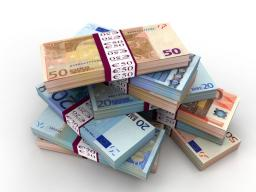Did you find an error or inaccuracy? Feel free to write us. Thank you!Tips to related online calculators

## Related math problems and questions:

• Bank deposit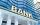Ms Jana after the withdraw amount of 2,500 euros after year saving in bank. What was her deposit if the interest rate was 2.5% p.a..
• BankPaul put 10000 in the bank for 6 years. Calculate how much you will have in the bank if he not pick earned interest or change deposit conditions. The annual interest rate is 3.5%, and the tax on interest is 10%.
• The depositDeposit has been placed for one year at an annual rate of 4.5%. After the added interst amount rose to € 2,612.5. Determine initial deposit.
• Millionaire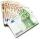Mr. Smith have gone withdraw from bank saving interest € 1,500. How big was his initial deposit if the annual interest rate is 1.5%?
• Deposit 3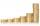After a year, Martin had on bank account € 2028. What was the interest rate when the initial deposit was € 1879?
• Profitable bank deposit 2012Calculate the value of what money lose creditor with a deposit € 9500 for 4 years if the entire duration is interest 2.6% p.a. and tax on interest is 19%. Annual inflation is 3.7% (Calculate what you will lose if you leave money lying idle at negative int
• Mr. Brown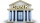Mr. Brown invested 15,000 euros in the bank for an annual term deposit. After the first year, he gained 600 euros. What was the annual interest rate of his deposit?
• Repay, interest, loanRamchacha takes a loan amount of 240000 from a bank for constructing a house at the rate of simple interest of 12% per annum. After 1 yr. of taking the loan he rents the house at the rate of 5200 per month. Determine the number of years he would take to r
• Rate or interestAt what rate percent will Rs.2000 amount to Rs.2315.25 in 3 years at compound interest?
• DepositIf you deposit 719 euros at the beginning of each year, how much money we have at 1.3% (compound) interest after 9 years?
• Compound interestCompound interest: Clara deposited CZK 100,000 in the bank with an annual interest rate of 1.5%. Both money and interest remain deposited in the bank. How many CZK will be in the bank after 3 years?
• Two accounts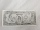Two accounts in the bank, one per year interest 2%, the second 3%. Total interest income 1900 USD. If interest rates were reversed, the yield would be USD 200 higher. What are the amounts on each account?
• SavingsThe depositor regularly wants to invest the same amount of money in the financial institution at the beginning of the year and wants to save 10,000 euros at the end of the tenth year. What amount should he deposit if the annual interest rate for the annua
• Bank account CZWe will deposit CZK 6,600 into the bank for 1 year with an interest rate of 2.5%. The interest tax is 15%. How many crowns will we receive after one year?
• Interst on savings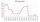The bank offers 1.6% interest. How many euros have to insert at the beginning if we received € 15 on the interest?
• When will I be a millionaire?Barry monthly send 280 euros to the bank, which he deposits bear interest of 2.1% p. A. Calculate how many months must Barry save to save 1000000 euros? Inflation, interest rate changes, or bank failures are ignored.
• Interest p.a.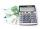Calculate the value of interest in period of 1 year from the deposit 62000 CZK at 8 percent interest rate, after deducting 15 percent tax.